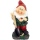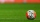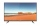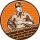# Algebra - math word problems

1. SevenSeven dwarfs will cut 420 stumps in 15 hours. After five hours, the two dwarves disappear discreetly. How many hours will the remaining dwarves complete the task?
2. StudentsStudents Aleš, Bohouš, Cyril, and Dušan were on a brigade. They divided the total revenue as follows: Aleš received two-fifths of the revenue, Bohouš received one-sixth of the revenue, Cyril received three-tenths of the revenue, and Dušan received the res
3. Job applicantsJob applicants: three-fourths of applicants had experience for a position. The number that did not have prior experience was 36. How many people applied for the job?
4. LibraryNew books were purchased for the library. Five-eighths were professional books, one-fifth were encyclopedias, and 231 books were dictionaries. How many professional books were there?
5. The basesThe bases of the isosceles trapezoid ABCD have lengths of 10 cm and 6 cm. Its arms form an angle α = 50˚ with a longer base. Calculate the circumference and content of the ABCD trapezoid.
6. What is bigger?Which ball has a larger volume: a football with a circumference of 66 cm or a volleyball with a diameter of 20 cm?
7. Double price reductionThe price of TV has been reduced twice. First by 15% and later by another 10% of the reduced price. After this double price reduction, the TV was sold for 8,874 crowns. What was the original price of the TV?
8. The angles ratioThe angles in the ABC triangle are in the ratio 1: 2: 3. find the sizes of the angles and determine what kind of a triangle it is.
9. Large familyThe average age of all family members (children, mother, father, grandmother, grandfather) is 29 years. The average age of parents is 40 years, grandparents 66 years and all children are 5 years. How many children are there in this family?
10. Equation with one variableSolve the following equation with one unknown: 5(7s + 5) =130
11. A mapA map with a scale of 1: 5,000 shows a rectangular field with an area of 18 ha. The length of the field is three times its width. The area of the field on the map is 72 cm square. What is the actual length and width of the field?
12. Water tank300hl of water was filled into the tank 12 m long and 6 m wide. How high does it reach?
13. BirthdaysIn the classroom, students always give candy to their classmates on their birthdays. The birthday person always gives each one candy, and he does not give himself. A total of 650 candies were distributed in the class per year. How many students are in the
14. Two trainsThe train runs at speed v1 = 72 km/h. The passenger, sitting in the train, observed that a train long l = 75m in 3 s passed on the other track in the opposite direction. Calculate the speed of this train.
15. Wallpaper3750 cm square of wallpaper is needed to glue a cube-shaped box. Can Dad cut out the whole necessary piece of wallpaper as a whole if he has a roll of wallpaper 50 cm wide?
16. Cone from cubeThe largest possible cone was turned from a 20 cm high wooden cube. Calculate its weight if you know that the density of wood was 850 kg/m3
17. Diameter = heightThe surface of the cylinder, the height of which is equal to the diameter of the base, is 4239 cm square. Calculate the cylinder volume.
18. Double probabilityThe probability of success of the planned action is 60%. What is the probability that success will be achieved at least once if this action is repeated twice?
19. BricklayersThe first bricklayer would build a wall in 3 days, the second in 4 days. The first bricklayer worked alone for a day, then the second bricklayer came to his aid and they finished the work together. How long did it take to build the wall?
20. The EarthThe Earth's surface is 510,000,000 km2. Calculates the radius, equator length, and volume of the Earth, assuming the Earth has the shape of a sphere.

Do you have an interesting mathematical word problem that you can't solve it? Submit math problem, and we can try to solve it.

We will send a solution to your e-mail address. Solved examples are also published here. Please enter the e-mail correctly and check whether you don't have a full mailbox.

Please do not submit problems from current active competitions such as Mathematical Olympiad, correspondence seminars etc...